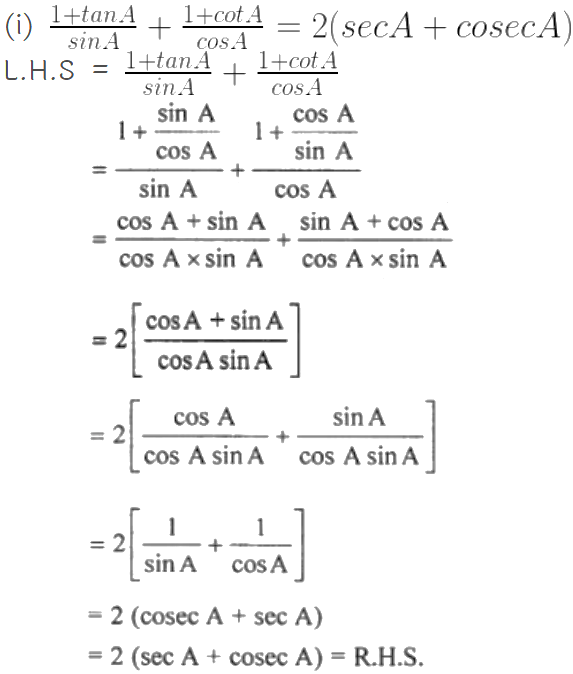Guru

# (i) (1 + tan A)/ sin A + (1 + cot A)/ cos A = 2 (sec A + cosec A) (ii) sec4 A – tan4 A = 1 + 2 tan2 A

• 0

This is an important question from the
Book- ML Aggarwal
Board- ICSE
Publication- Avichal
Chapter- Trigonometric Identities
Chapter number-18

We have to prove that

(i) (1 + tan A)/ sin A + (1 + cot A)/ cos A = 2 (sec A + cosec A) (ii) sec4 A – tan4 A = 1 + 2 tan2 A

Trigonometric Identities, class 10th, chapter 18th, question no 25, ICSE board, ML aggarwal..

Share

1. Solution:(ii) sec4 A – tan4 A = 1 + 2 tan2 A

L.H.S. = sec4 A – tan4 A

= (sec2 A – tan2 A) (sec2 A + tan2 A)

= (1 + tan4 A – tan4 A) (1 + tan4 A + tan4 A) [As sec2 A = tan4 A + 1]

= 1 (1 + 2 tan2 A)

= 1 + 2 tan2 A = R.H.S.

• 0